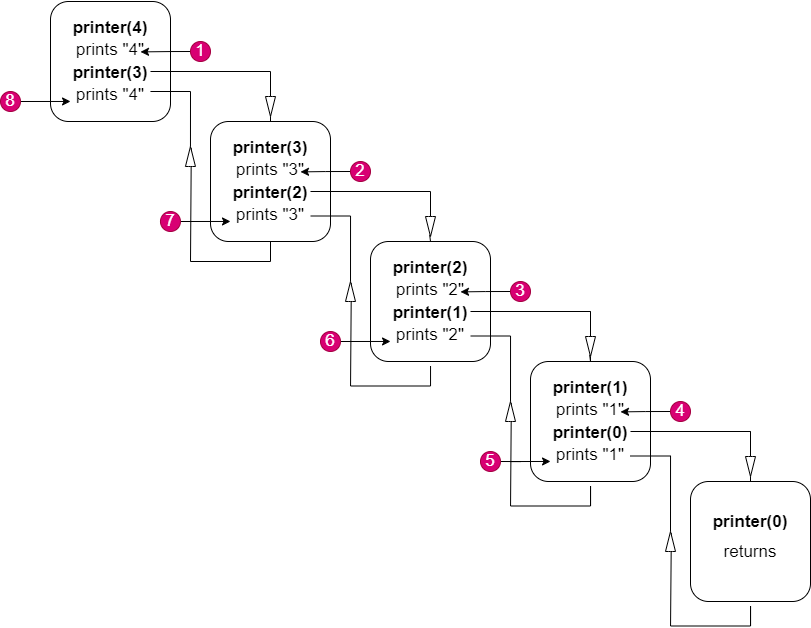# Print N to 1 and 1 to N Using Recursion

In this article we will learn how to solve the most asked coding interview problem: Print N to 1 and 1 to N Using Recursion.

Problem Statement: Given a number n. Print a sequence from n to 1 and again from 1 to n using recursion.

```Example:
Input:
n= 4

Output:
4 3 2 1 1 2 3 4
Explanation:
Since n is 4, the sequence starts from 4 to 1 and again from 1 to 4.
```

### Solution

Disclaimer: Don’t jump directly to the solution, try it out yourself first.

Intuition: We can simply have 2*n recursive calls to print 2*n numbers. But if we observe a recursive call carefully, we can print 2*n numbers in n recursive calls themselves. The intuition is to print the numbers above and below, the next recursive call. No need to memorize, we’ll see the approach below.

Approach:

• We have n, in order to print from n to 1, it is evident that at any function call, we can just print the number and hand over the control to the next recursive call.
• Let’s print from 1 to n. Just look from the perspective of a recursive function, it prints its parameter n, then the recursive call happens. When the call returns, we can assume that numbers from n-1 to 1 and 1 to n-1 are already printed, because of that call.
• Since we know until the recursive call returns, no code below the call executes, consider when we again print n(after the call), we can achieve what we need, right? By the time we are printing n for the 2nd time, numbers from n to 1 and 1 to n-1 are already printed.
• Have a look at the picture for better understanding. The number in the bubbles indicates the order, in which the numbers are printed.Code:

## C++ Code

``````#include<iostream>

using namespace std;

void printer(int n) {
if (n == 0) return;

cout << n << " ";
printer(n - 1);
cout << n << " ";
}

int main() {

int n = 4;
printer(n);

return 0;
}``````

Output: 4 3 2 1 1 2 3 4

Time Complexity:O(n).

Reason: Since the base condition is achieved only when n goes to 0, it takes n+1 recursive calls to reach 0.

Space Complexity: O(n).

Reason: We are using recursion which will internally use the stack. When n is at 0(n+1 recursive call), we would have made n recursive calls already and every function’s local variables are stored in the stack, which makes the linear space complex.

## Java Code

``````import java.util.*;

public class Solution {
public static void main(String[] args) {
int n = 4;
printer(n);
}

public static void printer(int n) {
if (n == 0) return;

System.out.print(n + " ");
printer(n - 1);
System.out.print(n + " ");
}
}``````

Output: 4 3 2 1 1 2 3 4

Time Complexity:O(n).

Reason: Since the base condition is achieved only when n goes to 0, it takes n+1 recursive calls to reach 0.

Space Complexity: O(n).

Reason: We are using recursion which will internally use the stack. When n is at 0(n+1 recursive call), we would have made n recursive calls already and every function’s local variables are stored in the stack, which makes the linear space complex.

Special thanks to Prathap P for contributing to this article on takeUforward. If you also wish to share your knowledge with the takeUforward fam, please check out this article